【一猫汽车网】全球顶级豪华SUV品牌路虎（Land Rover）用一场“征城挑战营”试驾体验活动让宾客们切身感受到了路虎品牌所蕴含的品牌精神。

【一猫汽车网】5月6日，上海。全球顶级豪华SUV品牌路虎（Land Rover）用一场“征城挑战营”试驾体验活动让宾客们切身感受到了路虎品牌所蕴含的“Above and Beyond”的品牌精神。活动期间，路虎品牌旗下两款国产明星座驾——发现神行和揽胜极光联袂登场，通过产品讲解、公路试驾及越野挑战等环节，全景展现了路虎车型始终如一的世界级品质和性能。此次路虎·发现神行&揽胜极光“征城挑战营”试驾体验活动将覆盖全国30个城市，旨在让更多消费者零距离体验路虎这两款各具特色SUV的独到魅力。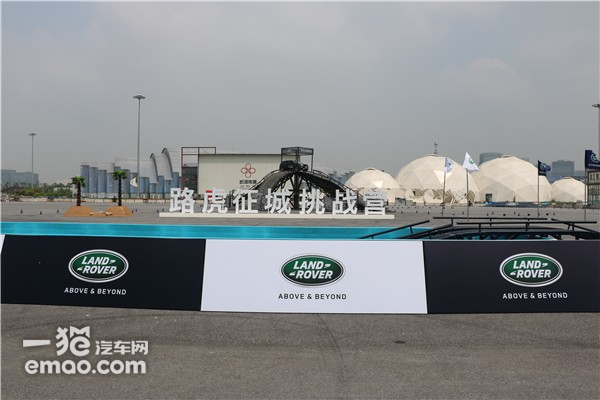试驾体验会上，除了发现神行和揽胜极光两款明星座驾外，路虎品牌精心设置的挑战项目也同样让人充满了征服欲，如高达6米、最大坡度为45°的Pagoda陡坡、30°极限侧坡、极具视觉冲击力的台阶路、高难度的坑洼路以及考验车辆涉水通过性的涉水路，均展示出了路虎产品无与伦比的越野性能。体验场外越野激情之余，来宾们也可在场内尽享路虎品牌所带来的尊崇体验。无论是专为儿童所设的趣野营吧，还是精致的剪纸艺术及小憩片刻的诱人美食都充分体现了路虎品牌的细致体贴和低调奢华。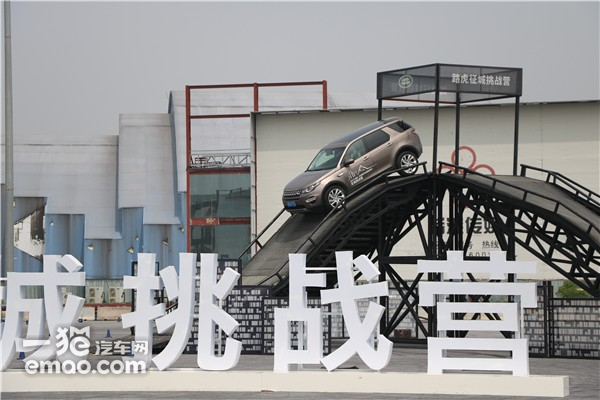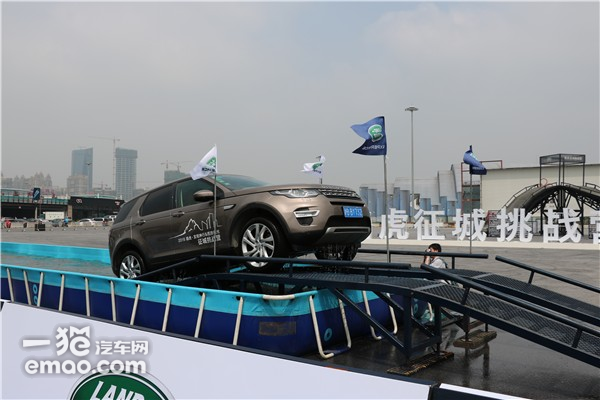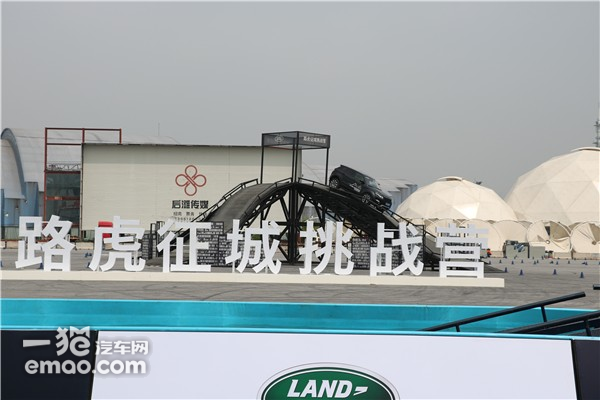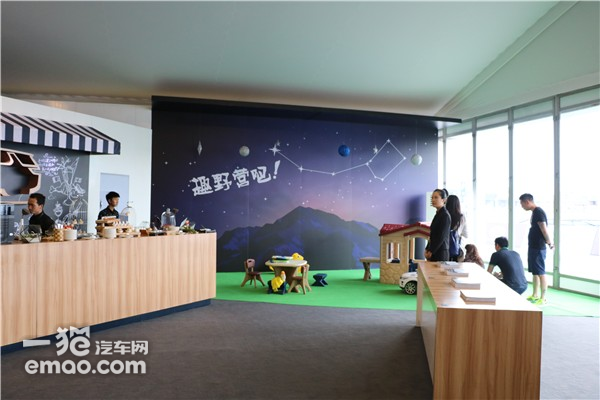发现神行是路虎发现家族全新推出的全能全地形豪华SUV车型，其卓越的越野和动力性能自然不在话下。在试驾过程中，让人印象更加深刻的是发现神行的多功能，尤其是其在同级车型中首创的“5+2”车内座椅配置，为消费者创造了一个灵活的空间组合：第二排座椅空间宽敞，还可前后灵活滑动160mm，实现更为舒适的空间体验，第三排座椅采用50:50分割设计，独立收放，折叠第二排与三排座椅可以提供1698升容积的后备箱空间，不论是载人还是载物都能自由组合，能充分满足全家休闲出行所需。同时，发现神行在全景天窗、车内影音娱乐、多个充电接口等细节上也应用了众多人性化设计，更让其全能性名副其实。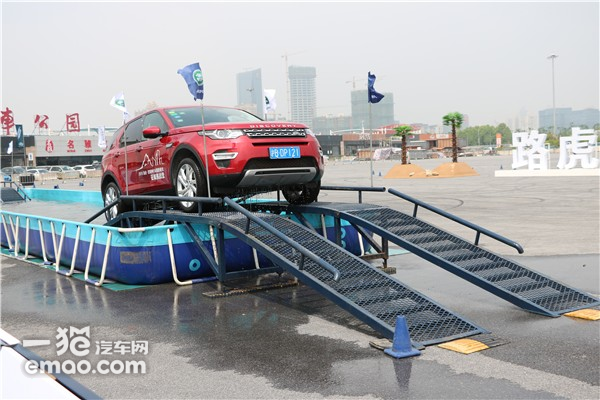而试驾体验的另一款明星车型——揽胜极光则代表了另外一种设计品位。魅力时尚的外观引领着都市潮流，让它成为了街头巷尾的风尚标。与耀目的外观相呼应，揽胜极光的内饰采用精挑细选的至臻材质，多重色彩搭配，多种时尚元素俱存，双缝线工艺与真皮座椅充分诠释揽胜家族的豪华理念，极致夺目，总耀不凡。当然，揽胜极光的这些高品质设计绝不是花架子，和发现神行一样，试驾过程中让人充分体验了其强大的动力与越野性能。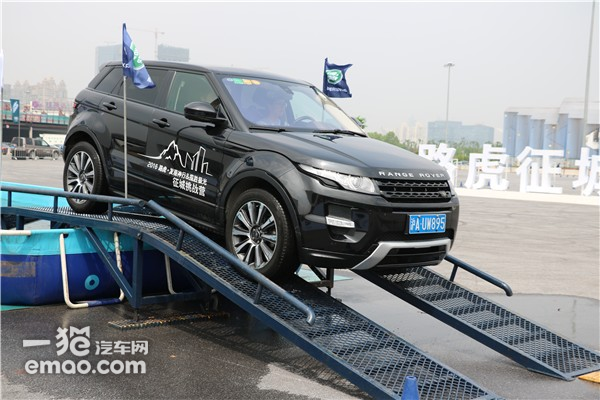发现神行和揽胜极光两款车型均搭载了240马力的2.0升Si4涡轮增压汽油发动机，可在每分钟1750转时达到峰值扭矩340牛·米，ZF 9速变速箱更可迅速细腻地换挡。在越野试驾项目体验上，路虎独有的全地形反馈适应系统可选择普通驾驶、草地/砂砾/雪地、泥沼/车辙和沙地四种模式，从容指挥车辆的马力及扭矩输出，轻松越过坑洼。下坡中，陡坡缓降控制系统让车辆以较低速度下坡，释放双脚，让人充分感受到路虎车型的极致驾控性能。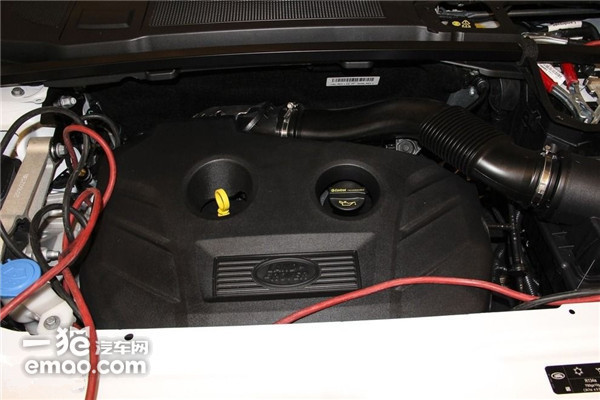作为路虎品牌在中国市场最先实现本地化生产的两款车型，发现神行和揽胜极光完美传承路虎品牌的纯正英伦血统，并集业界领先的前卫设计、精湛科技和卓越性能于一身。两款车均由奇瑞捷豹路虎常熟工厂出品，奇瑞捷豹路虎常熟工厂是捷豹路虎在英国以外的首个综合性整车制造基地，拥有世界一流的生产设施和严苛、完善的质量管理体系，被誉为“全球样板工厂”。奇瑞捷豹路虎常熟工厂于2012年11月奠基，2014年10月建成投产，创下了国内合资车企建设周期最短的纪录。诞生于信息和技术高度发达的工业4.0时代，奇瑞捷豹路虎常熟工厂在生产制造全过程中采用了大量业界领先的技术与设备，加之严苛、完善的质量管理体系，倾力打造“先进、高效、环保、品质”四大核心优势。无论是环境友好、节能减排的厂区布局设计，先进、高效的四大工艺流程，世界一流的质量中心和独有的车辆健康检查线，还是智能、高效的信息管理与物流系统，奇瑞捷豹路虎创立了诸多高端汽车卓越制造的样板，令业界瞩目。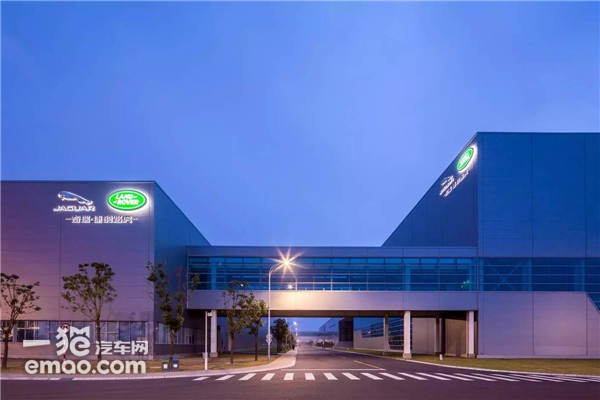试驾发现神行和揽胜极光，让人再次深刻感受到路虎“Above and Beyond”的品牌精神。这不仅体现在每一台路虎产品都可以让消费者彰显其独特、超凡的生活品味以及充满胆识、探险的探索精神，同时，路虎品牌追求旗下每一款车型在设计、科技、性能和品质上实现不断超越也淋漓尽致地体现了其自身品牌精神。

|分享

•已阅！握爪
•我手滑为你点赞
•128个赞！
•不明觉厉
•阅后既醉
•有钱！任性
•照片太美，我不敢看
•干货！杠杠的
•高大上
•膜拜中！
•请接受我的膝盖
•猫哥/猫妹么么哒
•神吐槽
•我只笑笑不说话
•我想静静
•窒息
##### 热度排行• 阿斯顿·马丁
• 奥迪
• 阿尔法罗密欧
• ALPINA
• 爱驰
• AC Schnitzer
• AITO
• ARCFOX极狐
• AUXUN傲旋

• 巴博斯
• 宝骏
• 宝马
• 保时捷
• 北汽制造
• 奔驰
• 奔腾
• 本田
• 比亚迪
• 标致
• 别克
• 宾利
• 布加迪
• 北汽威旺
• 北京
• 北汽绅宝
• 北汽幻速
• 北汽新能源
• 宝沃
• 比速汽车
• 北汽道达
• 比德文汽车
• 铂驰
• 北汽昌河
• 百智新能源
• 宝骐汽车
• 比克汽车
• 博速

• 昌河
• 长安
• 长城
• 长安商用
• 成功汽车
• 长江EV
• 长安轻车型
• 长安欧尚
• 长安凯程
• 车驰汽车
• 橙仕
• 创维汽车

• 大众
• 道奇
• 东风
• 东风风神
• 东风小康
• 东南
• DS
• 东风风行
• 东风风度
• 东风风光
• 电咖
• 大乘汽车
• 大运
• 东风富康
• 东风·瑞泰特
• 东风新能源
• 东风御风

• 法拉利
• 菲亚特
• 丰田
• 福迪
• 福特
• 福田汽车
• 福汽启腾
• 风诺
• 飞碟汽车
• 飞凡汽车

• GMC
• 广汽传祺
• 广汽吉奥
• 观致
• 国金汽车
• 国机智骏
• 高合汽车
• 广汽埃安

• 哈飞
• 海格
• 海马
• 华泰
• 黄海
• 恒天
• 红旗
• 哈弗
• 华颂
• 华凯
• 华泰新能源
• 汉腾汽车
• 华利
• 红星汽车
• HYCAN合创
• 汉龙汽车
• 恒润汽车
• 宏远汽车
• 华晨新日
• 华骐
• 华梓汽车

• Icona
• IMSA英飒

• Jeep
• 江淮
• 江铃
• 捷豹
• 金杯
• 九龙
• 吉利汽车
• 金旅
• 金龙
• 江铃集团轻汽
• 江铃集团新能源
• 君马汽车
• 捷途
• 钧天
• 捷达
• 几何汽车
• 极氪
• 捷尼赛思
• 金冠汽车

• 开瑞
• 凯迪拉克
• 科尼赛克
• 克莱斯勒
• KTM
• 卡威
• 凯翼
• 康迪
• 康迪电动汽车集团
• 开沃汽车
• 卡升
• 克蒂汽车
• 克慕勒

• 猎豹汽车
• 兰博基尼
• 劳斯莱斯
• 雷克萨斯
• 雷诺
• 理念
• 力帆
• 莲花汽车
• 林肯
• 铃木
• 陆风
• 路虎
• 路特斯
• 领志
• 领克
• 零跑汽车
• Lorinser
• LOCAL MOTORS
• 领途汽车
• 理想汽车
• 罗夫哈特
• 力帆汽车
• LEVC
• LIMGENE凌际
• LUMMA
• 莱茵汽车
• 岚图汽车
• 凌宝汽车
• 龙程汽车

• MG
• MINI
• 马自达
• 玛莎拉蒂
• 迈凯伦
• 摩根
• 迈莎锐
• 迈迈
• 摩登汽车

• 纳智捷
• 哪吒汽车

• 讴歌
• 欧宝
• 欧朗
• 欧拉
• 欧尚汽车

• 帕加尼
• Polestar极星
• 朋克汽车

• 奇瑞
• 启辰
• 起亚
• 前途
• 庆铃汽车
• 骐铃汽车
• 乔治·巴顿

• 日产
• 荣威
• 瑞麒汽车
• 如虎
• 瑞驰
• 瑞驰新能源
• 容大智造
• RAM
• 睿蓝汽车

### S

• 上汽大通
• smart
• 三菱
• 双环
• 双龙
• 斯巴鲁
• 斯柯达
• 萨博
• 思铭
• 赛麟
• SWM斯威汽车
• SRM鑫源
• 思皓
• SHELBY
• 上喆
• SERES赛力斯
• SONGSAN MOTORS
• 速达
• 申龙客车
• 神州

• TESLA
• 泰卡特
• 腾势
• 特斯拉
• 天际汽车
• TECHART
• 坦克

• 威麟
• 威兹曼
• 沃尔沃
• 五菱汽车
• 五十铃
• 潍柴英致
• WEY
• 蔚来
• 威马汽车
• WALD
• 瓦滋
• 伟昊汽车
• 魏牌

• 现代
• 雪佛兰
• 雪铁龙
• 西雅特
• 新特汽车
• 小鹏汽车
• 新宝骏
• 小虎
• 小猬汽车
• 晓奥汽车

• 野马汽车
• 一汽
• 依维柯
• 英菲尼迪
• 永源
• 驭胜
• 云度
• 宇通客车
• 云雀汽车
• 远程汽车
• 一汽凌河
• 雅升汽车
• 野马新能源
• 银隆新能源
• 御捷
• 裕路

• 中华
• 中兴
• 众泰
• 知豆
• 之诺
• 正道汽车
• 智点汽车
• 智己汽车
• 中国重汽VGV
• A
• B
• C
• D
• E
• F
• G
• H
• I
• J
• K
• L
• M
• N
• O
• P
• Q
• R
• S
• T
• U
• V
• W
• X
• Y
• Z

• 阿斯顿·马丁
• 奥迪
• 阿尔法罗密欧
• ALPINA
• 爱驰
• AC Schnitzer
• AITO
• ARCFOX极狐
• AUXUN傲旋

• 巴博斯
• 宝骏
• 宝马
• 保时捷
• 北汽制造
• 奔驰
• 奔腾
• 本田
• 比亚迪
• 标致
• 别克
• 宾利
• 布加迪
• 北汽威旺
• 北京
• 北汽绅宝
• 北汽幻速
• 北汽新能源
• 宝沃
• 比速汽车
• 北汽道达
• 比德文汽车
• 铂驰
• 北汽昌河
• 百智新能源
• 宝骐汽车
• 比克汽车
• 博速

• 昌河
• 长安
• 长城
• 长安商用
• 成功汽车
• 长江EV
• 长安轻车型
• 长安欧尚
• 长安凯程
• 车驰汽车
• 橙仕
• 创维汽车

• 大众
• 道奇
• 东风
• 东风风神
• 东风小康
• 东南
• DS
• 东风风行
• 东风风度
• 东风风光
• 电咖
• 大乘汽车
• 大运
• 东风富康
• 东风·瑞泰特
• 东风新能源
• 东风御风

• 法拉利
• 菲亚特
• 丰田
• 福迪
• 福特
• 福田汽车
• 福汽启腾
• 风诺
• 飞碟汽车
• 飞凡汽车

• GMC
• 广汽传祺
• 广汽吉奥
• 观致
• 国金汽车
• 国机智骏
• 高合汽车
• 广汽埃安

• 哈飞
• 海格
• 海马
• 华泰
• 黄海
• 恒天
• 红旗
• 哈弗
• 华颂
• 华凯
• 华泰新能源
• 汉腾汽车
• 华利
• 红星汽车
• HYCAN合创
• 汉龙汽车
• 恒润汽车
• 宏远汽车
• 华晨新日
• 华骐
• 华梓汽车

• Icona
• IMSA英飒

• Jeep
• 江淮
• 江铃
• 捷豹
• 金杯
• 九龙
• 吉利汽车
• 金旅
• 金龙
• 江铃集团轻汽
• 江铃集团新能源
• 君马汽车
• 捷途
• 钧天
• 捷达
• 几何汽车
• 极氪
• 捷尼赛思
• 金冠汽车

• 开瑞
• 凯迪拉克
• 科尼赛克
• 克莱斯勒
• KTM
• 卡威
• 凯翼
• 康迪
• 康迪电动汽车集团
• 开沃汽车
• 卡升
• 克蒂汽车
• 克慕勒

• 猎豹汽车
• 兰博基尼
• 劳斯莱斯
• 雷克萨斯
• 雷诺
• 理念
• 力帆
• 莲花汽车
• 林肯
• 铃木
• 陆风
• 路虎
• 路特斯
• 领志
• 领克
• 零跑汽车
• Lorinser
• LOCAL MOTORS
• 领途汽车
• 理想汽车
• 罗夫哈特
• 力帆汽车
• LEVC
• LIMGENE凌际
• LUMMA
• 莱茵汽车
• 岚图汽车
• 凌宝汽车
• 龙程汽车

• MG
• MINI
• 马自达
• 玛莎拉蒂
• 迈凯伦
• 摩根
• 迈莎锐
• 迈迈
• 摩登汽车

• 纳智捷
• 哪吒汽车

• 讴歌
• 欧宝
• 欧朗
• 欧拉
• 欧尚汽车

• 帕加尼
• Polestar极星
• 朋克汽车

• 奇瑞
• 启辰
• 起亚
• 前途
• 庆铃汽车
• 骐铃汽车
• 乔治·巴顿

• 日产
• 荣威
• 瑞麒汽车
• 如虎
• 瑞驰
• 瑞驰新能源
• 容大智造
• RAM
• 睿蓝汽车

### S

• 上汽大通
• smart
• 三菱
• 双环
• 双龙
• 斯巴鲁
• 斯柯达
• 萨博
• 思铭
• 赛麟
• SWM斯威汽车
• SRM鑫源
• 思皓
• SHELBY
• 上喆
• SERES赛力斯
• SONGSAN MOTORS
• 速达
• 申龙客车
• 神州

• TESLA
• 泰卡特
• 腾势
• 特斯拉
• 天际汽车
• TECHART
• 坦克

• 威麟
• 威兹曼
• 沃尔沃
• 五菱汽车
• 五十铃
• 潍柴英致
• WEY
• 蔚来
• 威马汽车
• WALD
• 瓦滋
• 伟昊汽车
• 魏牌

• 现代
• 雪佛兰
• 雪铁龙
• 西雅特
• 新特汽车
• 小鹏汽车
• 新宝骏
• 小虎
• 小猬汽车
• 晓奥汽车

• 野马汽车
• 一汽
• 依维柯
• 英菲尼迪
• 永源
• 驭胜
• 云度
• 宇通客车
• 云雀汽车
• 远程汽车
• 一汽凌河
• 雅升汽车
• 野马新能源
• 银隆新能源
• 御捷
• 裕路

• 中华
• 中兴
• 众泰
• 知豆
• 之诺
• 正道汽车
• 智点汽车
• 智己汽车
• 中国重汽VGV
• A
• B
• C
• D
• E
• F
• G
• H
• I
• J
• K
• L
• M
• N
• O
• P
• Q
• R
• S
• T
• U
• V
• W
• X
• Y
• Z

• 阿斯顿·马丁
• 奥迪
• 阿尔法罗密欧
• ALPINA
• 爱驰
• AC Schnitzer
• AITO
• ARCFOX极狐
• AUXUN傲旋

• 巴博斯
• 宝骏
• 宝马
• 保时捷
• 北汽制造
• 奔驰
• 奔腾
• 本田
• 比亚迪
• 标致
• 别克
• 宾利
• 布加迪
• 北汽威旺
• 北京
• 北汽绅宝
• 北汽幻速
• 北汽新能源
• 宝沃
• 比速汽车
• 北汽道达
• 比德文汽车
• 铂驰
• 北汽昌河
• 百智新能源
• 宝骐汽车
• 比克汽车
• 博速

• 昌河
• 长安
• 长城
• 长安商用
• 成功汽车
• 长江EV
• 长安轻车型
• 长安欧尚
• 长安凯程
• 车驰汽车
• 橙仕
• 创维汽车

• 大众
• 道奇
• 东风
• 东风风神
• 东风小康
• 东南
• DS
• 东风风行
• 东风风度
• 东风风光
• 电咖
• 大乘汽车
• 大运
• 东风富康
• 东风·瑞泰特
• 东风新能源
• 东风御风

• 法拉利
• 菲亚特
• 丰田
• 福迪
• 福特
• 福田汽车
• 福汽启腾
• 风诺
• 飞碟汽车
• 飞凡汽车

• GMC
• 广汽传祺
• 广汽吉奥
• 观致
• 国金汽车
• 国机智骏
• 高合汽车
• 广汽埃安

• 哈飞
• 海格
• 海马
• 华泰
• 黄海
• 恒天
• 红旗
• 哈弗
• 华颂
• 华凯
• 华泰新能源
• 汉腾汽车
• 华利
• 红星汽车
• HYCAN合创
• 汉龙汽车
• 恒润汽车
• 宏远汽车
• 华晨新日
• 华骐
• 华梓汽车

• Icona
• IMSA英飒

• Jeep
• 江淮
• 江铃
• 捷豹
• 金杯
• 九龙
• 吉利汽车
• 金旅
• 金龙
• 江铃集团轻汽
• 江铃集团新能源
• 君马汽车
• 捷途
• 钧天
• 捷达
• 几何汽车
• 极氪
• 捷尼赛思
• 金冠汽车

• 开瑞
• 凯迪拉克
• 科尼赛克
• 克莱斯勒
• KTM
• 卡威
• 凯翼
• 康迪
• 康迪电动汽车集团
• 开沃汽车
• 卡升
• 克蒂汽车
• 克慕勒

• 猎豹汽车
• 兰博基尼
• 劳斯莱斯
• 雷克萨斯
• 雷诺
• 理念
• 力帆
• 莲花汽车
• 林肯
• 铃木
• 陆风
• 路虎
• 路特斯
• 领志
• 领克
• 零跑汽车
• Lorinser
• LOCAL MOTORS
• 领途汽车
• 理想汽车
• 罗夫哈特
• 力帆汽车
• LEVC
• LIMGENE凌际
• LUMMA
• 莱茵汽车
• 岚图汽车
• 凌宝汽车
• 龙程汽车

• MG
• MINI
• 马自达
• 玛莎拉蒂
• 迈凯伦
• 摩根
• 迈莎锐
• 迈迈
• 摩登汽车

• 纳智捷
• 哪吒汽车

• 讴歌
• 欧宝
• 欧朗
• 欧拉
• 欧尚汽车

• 帕加尼
• Polestar极星
• 朋克汽车

• 奇瑞
• 启辰
• 起亚
• 前途
• 庆铃汽车
• 骐铃汽车
• 乔治·巴顿

• 日产
• 荣威
• 瑞麒汽车
• 如虎
• 瑞驰
• 瑞驰新能源
• 容大智造
• RAM
• 睿蓝汽车

### S

• 上汽大通
• smart
• 三菱
• 双环
• 双龙
• 斯巴鲁
• 斯柯达
• 萨博
• 思铭
• 赛麟
• SWM斯威汽车
• SRM鑫源
• 思皓
• SHELBY
• 上喆
• SERES赛力斯
• SONGSAN MOTORS
• 速达
• 申龙客车
• 神州

• TESLA
• 泰卡特
• 腾势
• 特斯拉
• 天际汽车
• TECHART
• 坦克

• 威麟
• 威兹曼
• 沃尔沃
• 五菱汽车
• 五十铃
• 潍柴英致
• WEY
• 蔚来
• 威马汽车
• WALD
• 瓦滋
• 伟昊汽车
• 魏牌

• 现代
• 雪佛兰
• 雪铁龙
• 西雅特
• 新特汽车
• 小鹏汽车
• 新宝骏
• 小虎
• 小猬汽车
• 晓奥汽车

• 野马汽车
• 一汽
• 依维柯
• 英菲尼迪
• 永源
• 驭胜
• 云度
• 宇通客车
• 云雀汽车
• 远程汽车
• 一汽凌河
• 雅升汽车
• 野马新能源
• 银隆新能源
• 御捷
• 裕路

• 中华
• 中兴
• 众泰
• 知豆
• 之诺
• 正道汽车
• 智点汽车
• 智己汽车
• 中国重汽VGV
• A
• B
• C
• D
• E
• F
• G
• H
• I
• J
• K
• L
• M
• N
• O
• P
• Q
• R
• S
• T
• U
• V
• W
• X
• Y
• Z